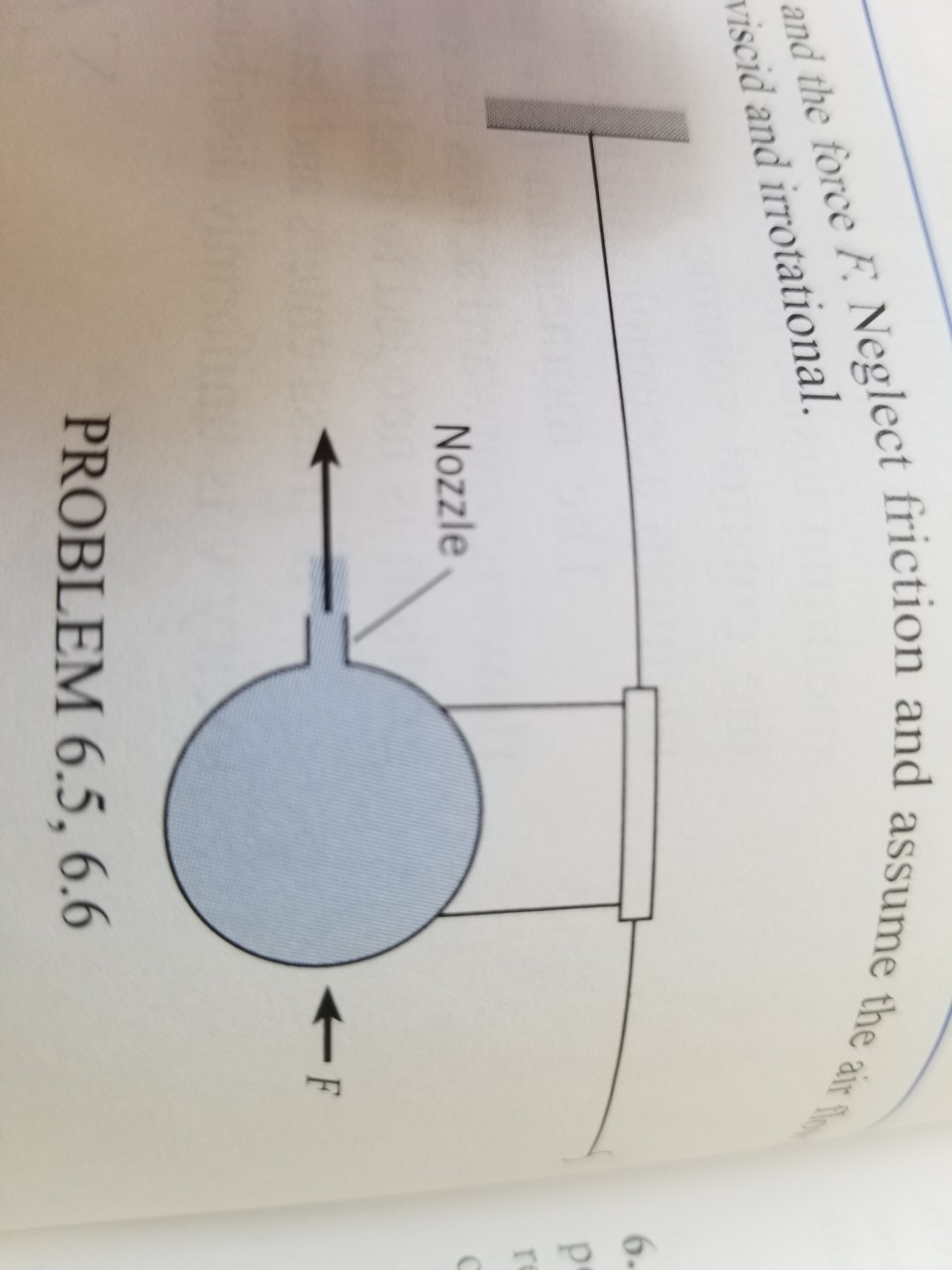# and the force F Neglect friction and assume the airviscid and irrotational6-rNozzleFPROBLEM 6.5, 6.6

Question

Fluid mechanics, jet problem:

The balloon rocket is held in place by a force, F. The pressure inside the balloon is 8 in-H2O, the nozzle diameter is 1.0 cm, and the air density is 1.2 kg/m3. Find the exit velocity, v and the force, F. Neglect friction and assume the air flow is inviscid and irrotational.help_outlineImage Transcriptioncloseand the force F Neglect friction and assume the air viscid and irrotational 6- r Nozzle F PROBLEM 6.5, 6.6 fullscreen
check_circleExpert Solution
Step 1

To determine: The exit velocity, v and the force  F.

Step 2

The pressure inside the balloon is 8 in-H2O.

Air density is 1.2kg/m3

The diameter of the nozzle, d is 1.0 cm

Step 3

Calculation:

The figure is shown...

### Want to see the full answer?

See Solution

#### Want to see this answer and more?

Solutions are written by subject experts who are available 24/7. Questions are typically answered within 1 hour*

See Solution
*Response times may vary by subject and question
Tagged in

### Fluid Mechanics